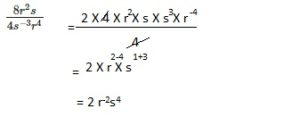# Math in Focus Grade 8 Chapter 1 Lesson 1.5 Answer Key Zero and Negative Exponents

Go through the Math in Focus Grade 8 Workbook Answer Key Chapter 1 Lesson 1.5 Zero and Negative Exponents to finish your assignments.

## Math in Focus Grade 7 Course 3 A Chapter 1 Lesson 1.5 Answer Key Zero and Negative Exponents

### Math in Focus Grade 8 Chapter 1 Lesson 1.5 Guided Practice Answer Key

Hands-On Activity

Understand the zero exponent

Work individually.

Step 1.
Use the power of a quotient property to simplify each expression. Write the quotient as an exponent.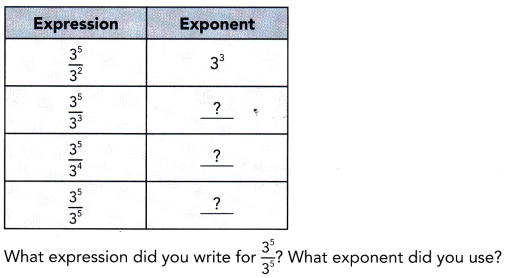Step 2.
In factored form, the quotient $$\frac{3^{5}}{3^{5}}$$ is $$\frac{3 \cdot 3 \cdot 3 \cdot 3 \cdot 3}{3 \cdot 3 \cdot 3 \cdot 3 \cdot 3}$$. If you divide out all the common factors in the numerator and denominator, what is the value of $$\frac{3^{5}}{3^{5}}$$?

Step 3.
Based on your findings, what can you conclude about the value of 3°?

Step 4.
Make a prediction about the value of any nonzero number raised to the zero power. Then, use a calculator to check your prediction for several numbers. For example, to raise the number —2 to the zero power, you can enter these keystrokes: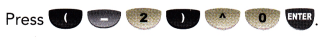Step 1: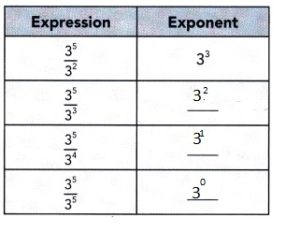Wrote the quotient as an exponent of 3° used exponent $$\frac{3^{5}}{3^{5}}$$,
Step 2:
Value is 1,
Step 3:
The value of 3° is 1.
Step 4:
Yes,

Explanation:
Step 1:
Used the power of a quotient property to simplify each expression.
Wrote the quotient as an exponent of 3° used exponent $$\frac{3^{5}}{3^{5}}$$,
Step2:
In factored form, the quotient $$\frac{3^{5}}{3^{5}}$$ is $$\frac{3 \cdot 3 \cdot 3 \cdot 3 \cdot 3}{3 \cdot 3 \cdot 3 \cdot 3 \cdot 3}$$. If you divide out all the common factors in the numerator and denominator the value of $$\frac{3^{5}}{3^{5}}$$ is 1 ,
Step 3 :
Any number to power 0 the value is 1, so the value of 3° is 1,
Step 4:
Makeing a prediction about the value of any nonzero number raised to the zero power.
Then, using a calculator to check your prediction for several numbers.
For example, to raise the number —2 to the zero power, you can enter these keystrokes:Yes my predictions hold true as
Any number to power 0 the value is 1.

Simplify each expression and evaluate where applicable.

Question 1.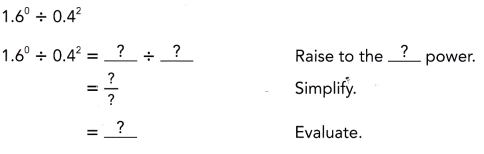(0.4)-2,

Explanation: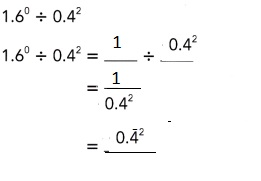Question 2.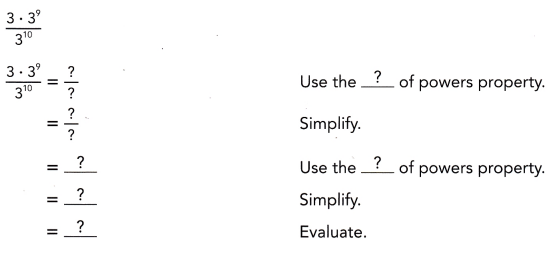1,

Explanation: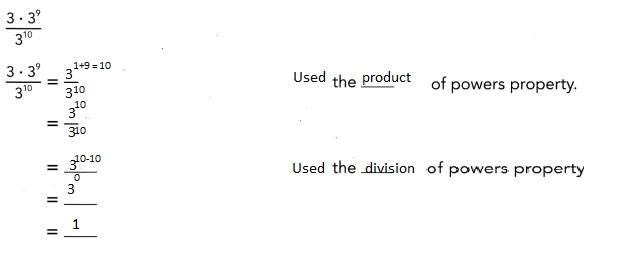Question 3.
$$\frac{t^{0} \cdot t^{7}}{t^{5}}$$
Alternatively, you can also apply the product of powers property to the expression t° ∙ t7 to get t0+7 = t7.
t2,

Explanation:
Given$$\frac{t^{0} \cdot t^{7}}{t^{5}}$$ as t0 is equal to 1  so
we get 1 X t7-5= t2.

Hands-On Activity

Work individually.

Step 1.
Use the quotient of powers property to simplify each expression. Write the quotient in exponential notation.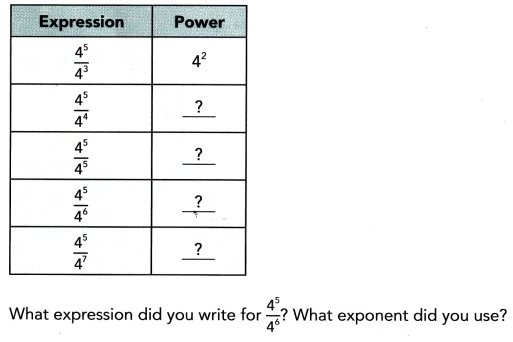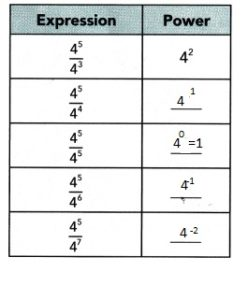Explanation:
Wrote the quotient in exponential notation above,
As (4)5÷ (4)6 = (4)5-6= (4)-1 used negative exponent.

Step 2.
In factored form, the quotient $$\frac{4^{5}}{4^{6}}$$ is $$\frac{4 \cdot 4 \cdot 4 \cdot 4 \cdot 4}{4 \cdot 4 \cdot 4 \cdot 4 \cdot 4 \cdot 4}$$. If you divide out all the common factors in the numerator and denominator, what is the value of
$$\frac{4^{5}}{4^{6}}$$?

The value is (4)-1,

Explanantion:
(4)5÷ (4)6 = (4)5-6= (4)-1 used negative exponent.

Step 3.
Repeat Step 2 for $$\frac{4^{5}}{4^{7}}$$. What is the value of $$\frac{4^{5}}{4^{7}}$$?

The value is (4)-2,

Explanantion:
(4)5÷ (4)7 = (4)5-7= (4)-2.

Math Journal
Suppose a represents any nonzero number. How would you write a-3 using a positive exponent?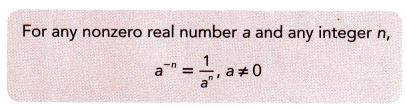Simplify each expression and evaluate where applicable.
1 ÷ a3 ,

Explanation:
Given  a-3 using a positive exponent for any nonzero real
number a and any integer 3, a-3 = 1 ÷ a3 ,a ≠ 0.

Question 4.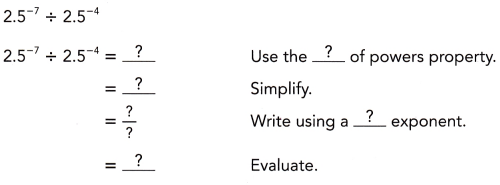(2.5)-3,

Explanantion: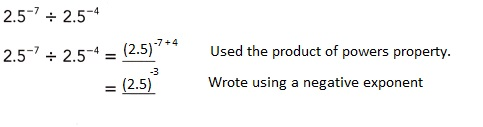Question 5.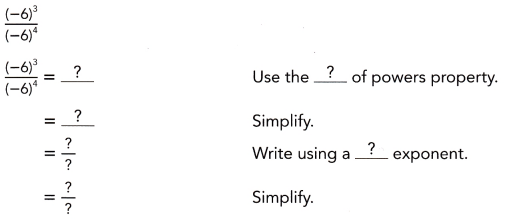(-6)-1,

Explanation: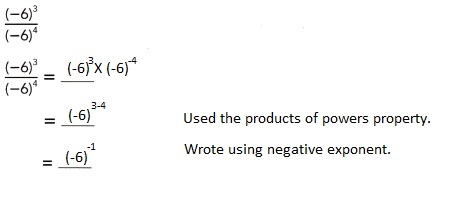Question 6.
14a-5 ÷ (7a • 2a-4)
14a-5 ÷ (7a • 2a-4) =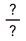Write the expression as a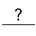.
=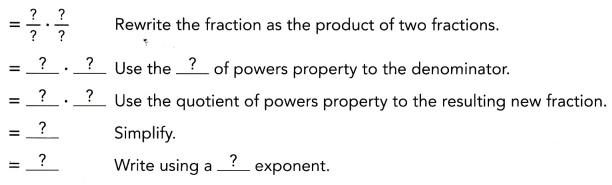1,

Explanation: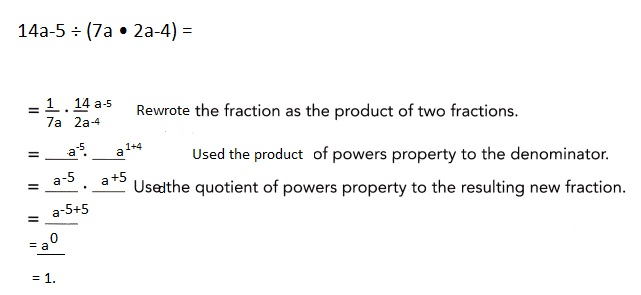unless asked to use a negative exponent.### Math in Focus Course 3A Practice 1.5 Answer Key

Simplify each expression and evaluate.

Question 1.
83 • 8°
83,

Explanation:
Given 83 • 8° any number to power 0 the value is 1, so the value of 8° is 1,
so 83 • 8° = 83 • 1 = 83.

Question 2.
54 • (-5)°
54,

Explanation:
Given 54 • (-5)° any number to power 0 the value is 1, so the value of (-5)° is 1,
so 54 • (-5)° = 54 • 1 = 54.

Question 3.
$$\left(\frac{1}{3}\right)^{4} \cdot\left(\frac{1}{3}\right)^{0}$$
$$\left(\frac{1}{3}\right)^{4}$$,

Explanation:
Given $$\left(\frac{1}{3}\right)^{4} \cdot\left(\frac{1}{3}\right)^{0}$$ as
$$\left(\frac{1}{3}\right)^{0}$$ = 1 therefore $$\left(\frac{1}{3}\right)^{4}$$ X 1 =
$$\left(\frac{1}{3}\right)^{4}$$.

Question 4.
7 • 103 + 42 • 102 + 5 • 10°
8,605,

Explanation:
Given 7 • 103 + 42 • 102 + 5 • 10° solving as 7 X 10 X 10 X 10 + 4 X 4 X 10 X 10 + 5 X 1 =
7 X 1,000 + 16 X 100 + 5 = 7,000 + 1,600 + 5 = 8,605.

Question 5.
(2.3) • 102 + 5 • 101 + 1 • 10°
281,

Explanation:
Given (2.3) • 102 + 5 • 101 + 1 • 10° solving as (2.3) X 10 X 10 + 5 X 10 + 1 X 1 =
230 + 50 + 1 =281.

Question 6.
$$\frac{7^{4} \cdot 7^{5}}{7^{9}}$$
1,

Explanation:
Given $$\frac{7^{4} \cdot 7^{5}}{7^{9}}$$ solving
$$\frac{7^{4+5}}{7^{9}}$$ = $$\frac{7^{9}}{7^{9}}$$=
(7)9-9 =(7)0 = 1.

Question 7.
(9-3)° • 52
52,

Explanation:
Given (9-3)° • 52 any number to power 0 the value is 1,
so the value of (9-3)°  is 1, therefore 1 X 52 = 52.

Question 8.
$$\frac{\left(6^{-3}\right)^{-2} \cdot 8^{6}}{48^{6}}$$
1

Explanation: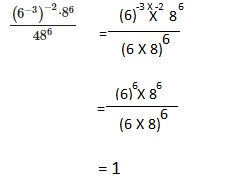Question 9.
73 • 7-4
7-1,

Explanation:
Given 73 • 7-4  using products of powers property we get
73-4 = 7-1.

Question 10.
$$\frac{(-5)^{-2}}{(-5)^{3}}$$
$$\frac{(-5)^{-2}}{(-5)^{3}}$$ simplify
= (-5)-1

Question 11.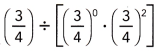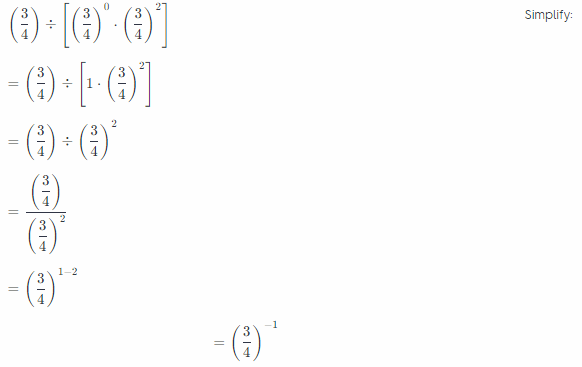Question 12.
$$\left(\frac{2}{5}\right)^{-4} \cdot\left(\frac{2}{5}\right)^{-1} \div\left(\frac{2}{5}\right)^{-3}$$
$$\left(\frac{2}{5}\right)^{-2}$$,

Explanation: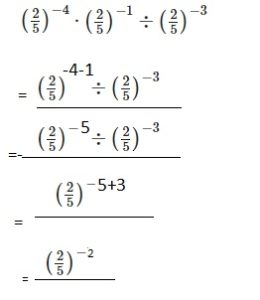Question 13.
$$\frac{x^{0}}{x^{2} \cdot x^{3}}$$
x -5,

Explanation: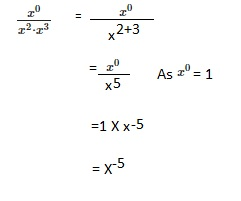Question 14.
$$\frac{4 h^{-5} \cdot 6 h^{-2}}{3 h^{-3}}$$
8(h)-4,

Explanation: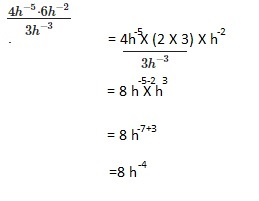Question 15.
1.2° ÷ 1.82
0.308,

Explanation:
Given 1.2° ÷ 1.82 any number to power 0 the value is 1,
so the value of (1.2)° is 1, therefore 1÷1.82 = 1 ÷ 3.24 = 0.308.

Question 16.
5.2-3 ÷ 2.6-3
0.125,

Explanation:
Given 5.2-3 ÷ 2.6-3  we get  2.63 ÷ 5.23 upon solving
(2.6 X 2.6 X 2.6) ÷ (5.2 X 5.2 X 5.2) = 0.125.

Question 17.
$$\frac{(-3)^{-4}}{(-3)^{2}}$$
$$\frac{(1)}{(-3)^{6}}$$,

Explanation:
Given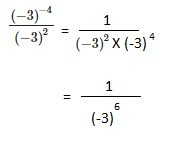Question 18.
$$\left(\frac{5}{6}\right)^{-4} \cdot\left(\frac{5}{6}\right)^{-2} \div\left(\frac{5}{6}\right)^{-3}$$
$$\frac{(1)}{\frac{5}{6}^{3}}$$,

Explanation: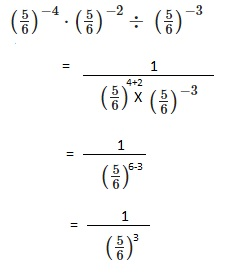Question 19.
$$\frac{9 k^{-1} \cdot 2 k^{-3}}{27 k^{-6}}$$
2k2 ÷ 3,

Explanation: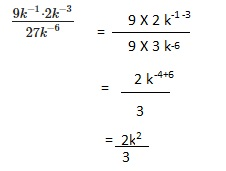Question 20.
$$\frac{c^{-4} \cdot c^{12}}{c^{-7}}$$
(c)15,

Explanation: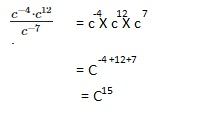Evaluate each numeric expression.

Question 21.
$$\frac{7^{-2} \cdot 7^{0}}{8^{3} \cdot 8^{-5}}$$
(8/7)2,

Explanation: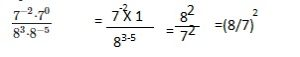Question 22.
$$\frac{\left(7^{-2}\right)^{2} \cdot 9^{-4}}{21^{-4}}$$
(3)-4,

Explanation: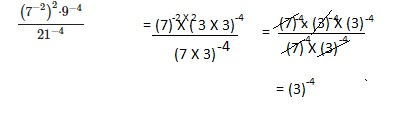Question 23.
$$\frac{10^{0}}{2^{-2} \cdot\left(5^{-1}\right)^{2}}$$
100,

Explanation: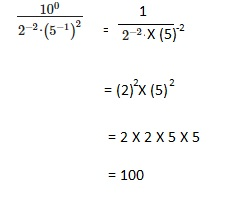Question 24.
$$\frac{\left(3^{6}\right)^{-2}}{6^{-9}\left(-2^{10}\right)}$$
(-1/54),

Explanation: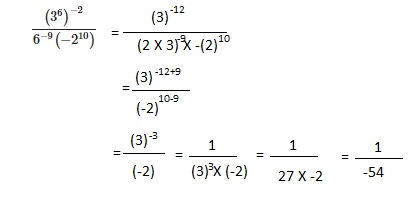Simplify each algebraic expression.

Question 25.
$$\left(\frac{7 m^{3}}{-49 n^{0}}\right)^{-1}$$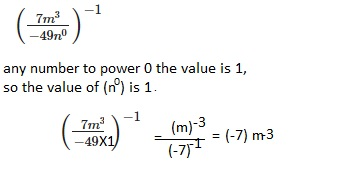$$\frac{8 r^{2} s}{4 s^{-3} r^{4}}$$